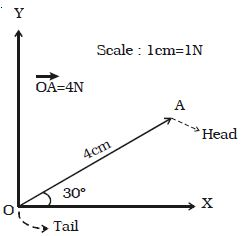Physics

# Representation of Vector

Vector quantifies are often represented by a scaled vector diagrams. Vector diagrams represent a vector by the use of an arrow drawn to scale in a specific direction. An example of a scaled vector diagram is shown in figure.From the figure, it is clear that

(i) The scale is listed.

(ii) A line with an arrow is drawn in a specified direction.

(iii) The magnitude and direction of the vector are clearly labelled. In the above case, the diagram shows that the magnitude is 4 N and direction is 30° to x-axis. The length of the line gives the magnitude and arrow head gives the direction. In notation, the vector is denoted in bold face letter such as A or with an arrow above the Ǡ letter as A. read as vector A or A vector while its magnitude is denoted by A or by │Ǡ│.